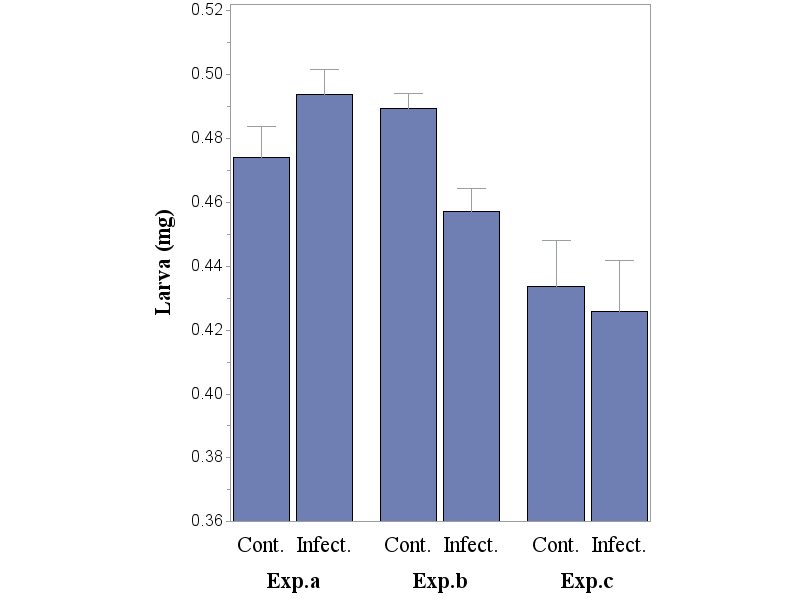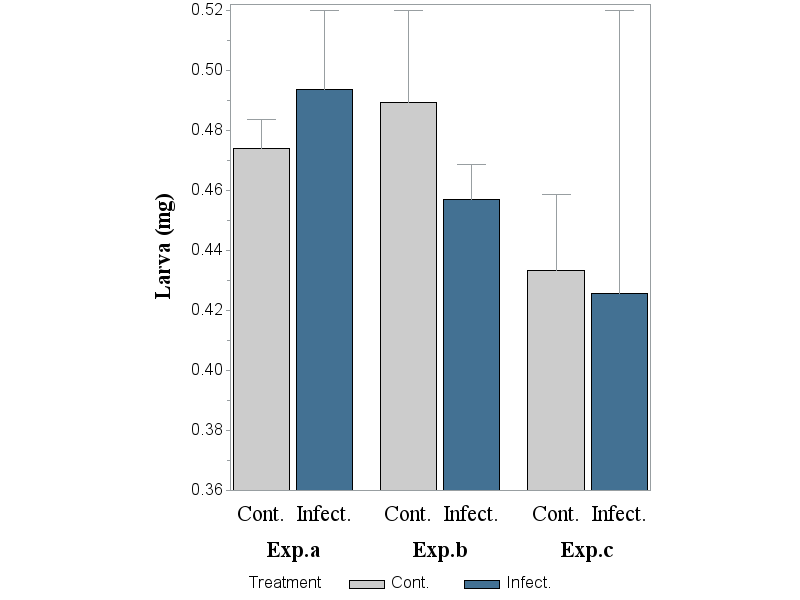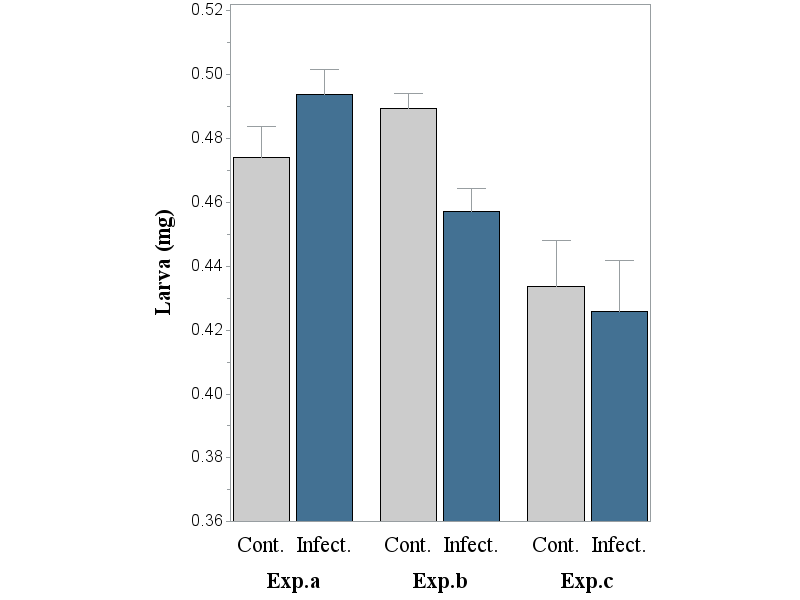## proc gchart: adding 'subgroup' gives different error bars

Dear all,

When adding 'subgroup' to the gchart options, in order to allow bars have pre-defined patterns, the error bars are suddenly wrongly calculated. I do not know what the reason would be, and how to solve the problem for this simple graph...

All help more than welcome

(A) below the code and barchart without subgroup, and correctly calculated error bars:goptions reset=all;
proc gchart data = cornell.transweightfinalchart;
legend label=(h=1.5) VALUE=(h=1.5);
axis1 label=(f='Times new roman/bo' h=2 a=90 "Larva (mg)" ) order=(0.36 to 0.52 by 0.02) value=(h=1.5) minor=(n=1);
axis2 label=none value=(h=2 f='Times new roman');
axis3 label=none value=(h=2 f='Times new roman/bo');
vbar Treatment/
group=Experiment
sumvar=L
errorbar=top
clm=68.26
width= 8
space= 1
gspace= 3
legend= legend
type=mean
raxis= axis1
maxis=axis2
gaxis=axis3;
where treatment ne '';
run;
quit;

(B) Below a subgroup, named 'Treatment' is added in order to adjust the pattern of the bar that represents either the the Cont. or the Infect. group (i.e. also pattern1 and pattern2 has been added). Error bars: not correctgoptions reset=all;
proc gchart data = cornell.transweightfinalchart;
pattern1 v=solid color=graycc;
pattern2 v=solid color=cx437193;
legend label=(h=1.5) VALUE=(h=1.5);
axis1 label=(f='Times new roman/bo' h=2 a=90 "Larva (mg)" ) order=(0.36 to 0.52 by 0.02) value=(h=1.5) minor=(n=1);
axis2 label=none value=(h=2 f='Times new roman');
axis3 label=none value=(h=2 f='Times new roman/bo');
vbar Treatment/
group=Experiment
sumvar=L
errorbar=top
clm=68.26
subgroup=Treatment
width= 8
space= 1
gspace= 3
legend= legend
type=mean
raxis= axis1
maxis=axis2
gaxis=axis3;
where treatment ne '';
run;
quit;

What did I do wrong?

2 REPLIES 2DanH_sas
SAS Super FREQ

## Re: proc gchart: adding 'subgroup' gives different error bars

Take away the SUBGROUP and add PATTERNID=MIDPOINT to see if you get what you expect.

Hope this helps!

Dan

## Re: proc gchart: adding 'subgroup' gives different error bars

Wow Dan,

this is THE solution, indeed!  I do not know why, but it worked.

Thank you so much!

DieterDiscussion stats
• 2 replies
• 235 views
• 1 like
• 2 in conversation